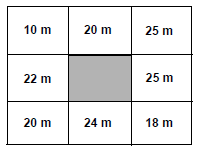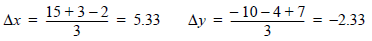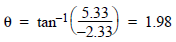# Aspect Images

## Producer Field Guide

HGD_Product
Producer Field Guide
HGD_Portfolio_Suite
Producer

An aspect image is an image file that is gray scale coded according to the prevailing direction of the slope at each pixel. Aspect is expressed in degrees from north, clockwise, from 0 to 360. Due north is 0 degrees. A value of 90 degrees is due east, 180 degrees is due south, and 270 degrees is due west. A value of 361 degrees is used to identify flat surfaces such as water bodies.

Use Aspect function to generate an aspect image.

As with slope calculations, aspect uses a 3 × 3 window around each pixel to calculate the prevailing direction it faces. For pixel X,Y containing the following elevation values around it, the average changes in elevation in both x and y directions are calculated first. In this figure, Pixel X,Y has elevation value e. The pixels around Pixel X,Y in a 3 x 3 window have the elevations a, b, c, d, e, f, g, h, and i. Each pixel has a ground resolution of 30 × 30 meters.

3 × 3 Window Calculates the Aspect at Each Pixel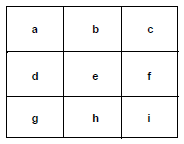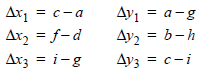Where:

a...i = elevation values of pixels in a 3 × 3 window as shown above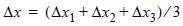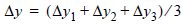If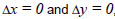then the aspect is flat (coded to 361 degrees). Otherwise,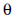is calculated as: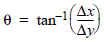Note thatis calculated in radians.

Then, aspect is 180 +(in degrees).

Example

Aspect files are used in many of the same applications as slope files. In transportation planning, for example, north facing slopes are often avoided. Especially in northern climates, these would be exposed to the most severe weather and would hold snow and ice the longest. It would be possible to recode all pixels with north facing aspects as undesirable for road building.

A hypothetical example is given in the figure below, showing how the aspect is calculated for a single pixel. The shaded pixel is the pixel for which slope is being calculated. The elevations of neighboring pixels are expressed in meters.

Aspect Calculation Example